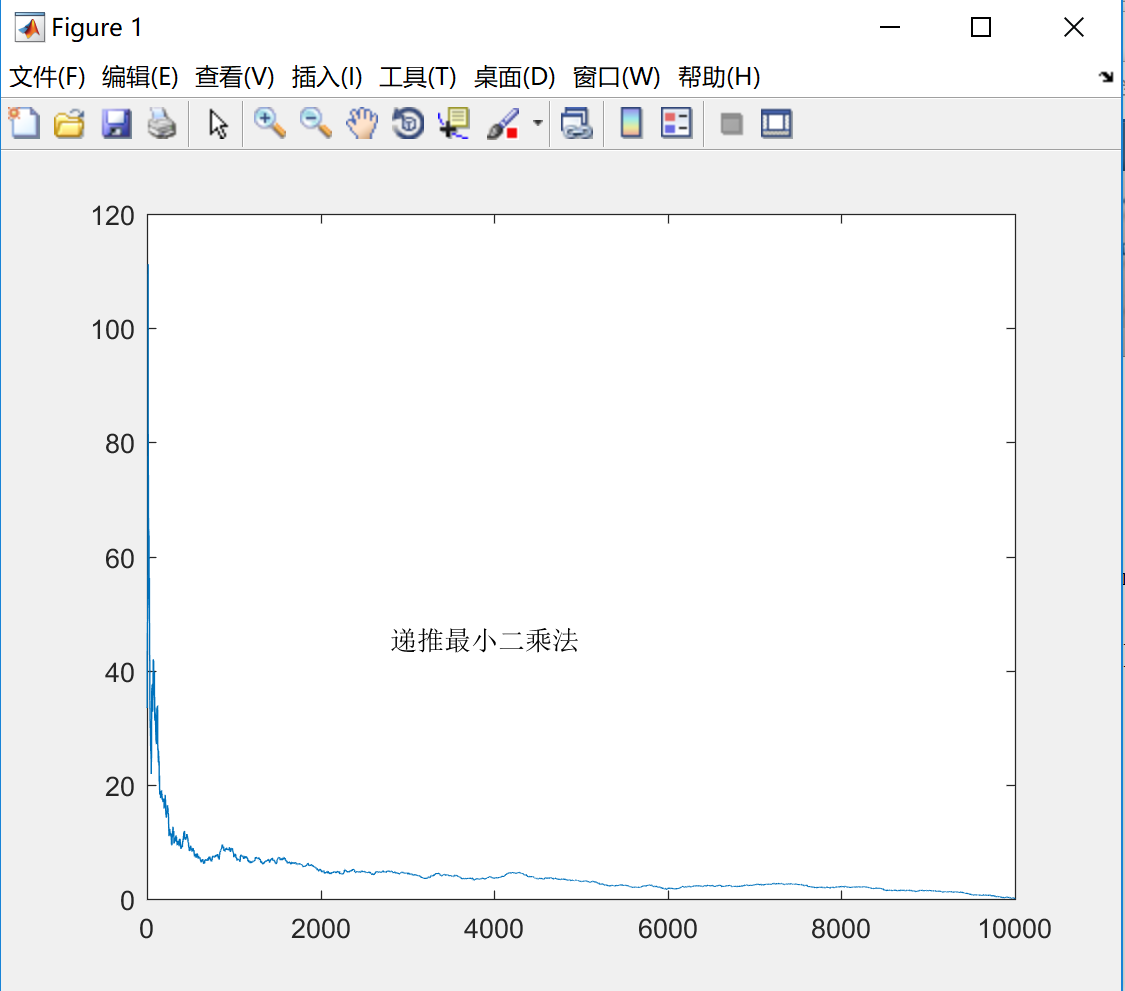# Halo

A magic place for coding

0%

## 介绍

我们在以前可能会在直线拟合的时候使用过最小二乘法，常用于处理实现数据，为了获得一条直线或者曲线。而在这里我们对更一般的情况做讨论。
对于给定的超定线性方程组$Ax = b$，直接通过矩阵求解是困难的。这里就用到了逼近的思想。假设上述方程存在一个噪声$e$，则可以写成$b-Ax = e$，接下来要做的就是求解出能够极小化这个噪声的$x$。这就是递推最小二乘法需要解决的问题。

## 分析

递推最小二乘法的思路是基于最小二乘法，采用递推的形式来求得最终的$x$。首先来分析$x$的形式：
$$x^* = \min_x ||b-Ax||^2，$$

$$2A^T(Ax - b) = 0 \ x = (A^TA)^{-1}A^Tb$$

$$K(m) = \frac{P(m-1)\phi_m}{1+\phi_m^TP(m-1)\phi_m} \ P(m) = (I-K(m)\phi_m^T)P(m-1) \ \hat x(m) = \hat x(m-1) + K(m)(b_m - \phi_m^T\hat x(m-1))$$
，其中$\phi_m^T$为矩阵$A$的第$m$行。
每一步迭代，我们都通过在旧的估计值上，加上经过预测误差的真正测量值来得到新的估计值，最后得到较为准确的结果$x$。

## 代码实现

根据上述推导的公式，编写matlab代码，特别需要注意$\phi_m^T$是$A(m,:)$，而$\phi_m$是$A(m,:)’$。

## 小结

实现并运行上述代码，进行简单的数值测试。递推最小二乘法的分析与实现就到这里了，谢谢！

#### 参考资料：

1.数值分析（第5版） 李庆扬，王能超，易大义

Welcome to my other publishing channels